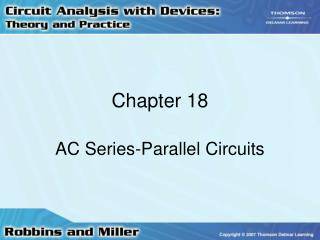Download PresentationChapter 18

Chapter 18 - PowerPoint PPT Presentation

Chapter 18. AC Series-Parallel Circuits. AC Circuits. Rules and laws developed for dc circuits apply equally well for ac circuits Analysis of ac circuits requires vector algebra and use of complex numbers Voltages and currents in phasor form Expressed as RMS (or effective) values. Ohm’s Law.I am the owner, or an agent authorized to act on behalf of the owner, of the copyrighted work described.
Download PresentationChapter 18

Download Policy: Content on the Website is provided to you AS IS for your information and personal use and may not be sold / licensed / shared on other websites without getting consent from its author.While downloading, if for some reason you are not able to download a presentation, the publisher may have deleted the file from their server.

- - - - - - - - - - - - - - - - - - - - - - - - - - E N D - - - - - - - - - - - - - - - - - - - - - - - - - -
Presentation Transcript
1. Chapter 18 AC Series-Parallel Circuits

2. AC Circuits • Rules and laws developed for dc circuits apply equally well for ac circuits • Analysis of ac circuits requires vector algebra and use of complex numbers • Voltages and currents in phasor form • Expressed as RMS (or effective) values

3. Ohm’s Law • Voltage and current of a resistor will be in phase • Impedance of a resistor is: ZR = R0°

4. Ohm’s Law • Voltage across an inductor leads the current by 90°(ELI the ICE man)

5. Ohm’s Law • Current through a capacitor leads the voltage by 90° (ELI the ICE man)

6. AC Series Circuits • Current everywhere in a series circuit is the same • Impedance used to collectively determine how resistance, capacitance, and inductance impede current in a circuit

7. AC Series Circuits • Total impedance in a circuit is found by adding all individual impedances vectorially

8. AC Series Circuits • Impedance vectors will appear in either the first or the fourth quadrants because the resistance vector is always positive • When impedance vector appears in first quadrant, the circuit is inductive

9. AC Series Circuits • If impedance vector appears in fourth quadrant • Circuit is capacitive

10. Voltage Divider Rule • Voltage divider rule works the same as with dc circuits • From Ohm’s law:

11. Kirchhoff’s Voltage Law • KVL is same as in dc circuits • Phasor sum of voltage drops and rises around a closed loop is equal to zero

12. Kirchhoff’s Voltage Law • Voltages • May be added in phasor form or in rectangular form • If using rectangular form • Add real parts together • Then add imaginary parts together

13. AC Parallel Circuits • Conductance, G • Reciprocal of the resistance • Susceptance, B • Reciprocal of the reactance

14. AC Parallel Circuits • Admittance, Y • Reciprocal of the impedance • Units for all of these are siemens (S)

15. AC Parallel Circuits • Impedances in parallel add together like resistors in parallel • These impedances must be added vectorially

16. AC Parallel Circuits • Whenever a capacitor and an inductor having equal reactances are placed in parallel • Equivalent circuit of the two components is an open circuit

17. Kirchhoff’s Current Law • KCL is same as in dc circuits • Summation of current phasors entering and leaving a node • Equal to zero

18. Kirchhoff’s Current Law • Currents must be added vectorially • Currents entering are positive • Currents leaving are negative

19. Current Divider Rule • In a parallel circuit • Voltages across all branches are equal

20. Series-Parallel Circuits • Label all impedances with magnitude and the associated angle • Analysis is simplified by starting with easily recognized combinations

21. Series-Parallel Circuits • Redraw circuit if necessary for further simplification • Fundamental rules and laws of circuit analysis must apply in all cases

22. Frequency Effects of RC Circuits • Impedance of a capacitor decreases as the frequency increases • For dc (f = 0 Hz) • Impedance of the capacitor is infinite

23. Frequency Effects of RC Circuits • For a series RC circuit • Total impedance approaches R as the frequency increases • For a parallel RC circuit • As frequency increases, impedance goes from R to a smaller value

24. Frequency Effects of RL Circuits • Impedance of an inductor increases as frequency increases • At dc (f = 0 Hz) • Inductor looks like a short • At high frequencies, it looks like an open

25. Frequency Effects of RL Circuits • In a series RL circuit • Impedance increases from R to a larger value • In a parallel RL circuit • Impedance increases from a small value to R

26. Corner Frequency • Corner frequency is a break point on the frequency response graph • For a capacitive circuit • C = 1/RC = 1/ • For an inductive circuit • C = R/L = 1/

27. RLC Circuits • In a circuit with R, L, and C components combined in series-parallel combinations • Impedance may rise or fall across a range of frequencies • In a series branch • Impedance of inductor may equal the capacitor

28. RLC Circuits • Impedances would cancel • Leaving impedance of resistor as the only impedance • Condition is referred to as resonance

29. Applications • AC circuits may be simplified as a series circuit having resistance and a reactance • AC circuit • May be represented as an equivalent parallel circuit with a single resistor and a single reactance

30. Applications • Any equivalent circuit will be valid only at the given frequency of operation